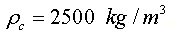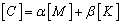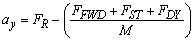Shahriar Abtahi, Parviz Ghadimi, Rahim Zamanian, Seye Reza DjeddiOPEN ACCESSPEER-REVIEWED

Shahriar Abtahi1, Parviz Ghadimi1, Rahim Zamanian2,, Seye Reza Djeddi1

1Department of Marine Technology, Amirkabir University of Technology, Iran

2International Campus-Mech. Eng’g. Group, Amirkabir University of Technology, Iran

### Abstract

Optimal design of Quay Walls against seismic forces has become a challenge for engineers. For countries that are placed on the earthquake belt, it is very important to investigate the strength and behavior of quay walls against earthquakes. In this study, the behavior of cantilever concrete quay walls placed on the saturated cohesive soil is studied using finite element method in ANSYS Software. Patterns of the interaction between soil and structure prove to be very complicated. On the other hand, with the consideration of the interactions between soil and structure under the seismic forces, the problem will be made much more complicated. In the present study, height of the quay walls, soil type, strength and duration of the earthquake on the Bending moment against the wall are considered and pressure distributions behind the wall have been investigated.

### At a glance: Figures

• Abtahi, Shahriar, et al. "Investigation of Soil and Quay Wall Interaction Under Seismic Loading." American Journal of Civil Engineering and Architecture 1.3 (2013): 52-58.
• Abtahi, S. , Ghadimi, P. , Zamanian, R. , & Djeddi, S. R. (2013). Investigation of Soil and Quay Wall Interaction Under Seismic Loading. American Journal of Civil Engineering and Architecture, 1(3), 52-58.
• Abtahi, Shahriar, Parviz Ghadimi, Rahim Zamanian, and Seye Reza Djeddi. "Investigation of Soil and Quay Wall Interaction Under Seismic Loading." American Journal of Civil Engineering and Architecture 1, no. 3 (2013): 52-58.

 Import into BibTeX Import into EndNote Import into RefMan Import into RefWorks

### 1. Introduction

Quay walls are utilized to protect the coastal line in particular areas. One of the methods which could be used to analyze the loads caused by Seismic forces against the quay walls is the numerical method. In this type of modeling, interactions between soil and structure are investigated. In the modeling process, if the soil stretches long enough and far from the structure in order to reduce the finite effect of the computational domain, the considered model would be more accurate and, compared with other methods, would be much closer to the reality.

Classic methods for seismic analyses of quay walls have been implemented by Futaki , Seed and Whitman  and Rowe . Also, substrate reaction method has been exerted by Winkler et.al. , Terzaghi  and Dewaikar and Halkude . In 1986 and afterwards in 1995, Halliburton  and Simon  have used this method for the analysis of the quay walls, respectively. Experimental methods were first introduced by Terzaghi  despite the fact that their computational cost would be much higher in comparison to other methods. Later, Peck [10, 11] used the experimental methods for analyzing the problem at hand. Finally with the introduction of Finite Element Methods (FEM) and based on the accuracy and efficiency of this numerical tool, Clough  introduced the use of numerical modeling for this problem. The basis and criteria for the design of the quay wall was checked and investigated by Goda . In the recent years, Munireddy  investigated the pressure reduction methods due to the wave impact on the quay caisson walls. Based on the investigations made on the current literature, it is clear that there has been less focus on the modeling of three different phases (Water, Quay Wall and Soil) altogether. Also previous works have been majorly focused on the static interaction of water while in the present study the aim is to model the three phase interaction problem using dynamic modeling of fluid effects. It is worth mentioning that some of the previous studies have assumed the dynamic behavior of water [13, 14] while in these works the soil is assumed to be rigid or replaced by a fixed displacement. Therefore, in the present study the deformable or flexible soil is used instead of the rigid material to fully model the three-phase interaction problem.

In the present study, based on the assumption of the elastic behavior of the quay walls, the effect of the height, type and rigidity of the walls on this behavior and the distribution of the pressure and respective anchor are studied and investigated.

### 2. Modeling Procedure

In the present work, modeling of the soil and structure is done using a direct method with the consideration of boundary and initial conditions, respectively. The steel walls with respective elastic properties are modeled and the automatic “beam” elements has been used in order to generate mesh from the geometrical domain. Three dimensional Moher Coloumb elements  with eight nodes were also used for the meshing of the soil material. In the framework of ANSYS Software, meshing of the fluid domain in three dimensional is done based on suitable fluid elements, and interface elements were used for the region of fluid-structure interaction. Moreover, in order to reduce the reflection of the earthquake waves from the boundaries, the length of the computational domain is increased. This reflection is decreased even more with the addition of absorbing boundary to the present model. The computation domain and the generated mesh are depicted in Figure 1, Figure 2, respectively.

A variety of Eigen solvers are available in ANSYS for the analysis and calculation of various modes and Eigen-values. Each solver has its own preferences based on the computational speed and computational cost, number of the required frequencies and also the accuracy of the obtained results. In the case of the present problem, based on the required solution outputs, the Full Method is used. The accuracy of this method depends on the Multi Degree of Freedom (MDOF) mode that is selected by the model. These modes are the most important parameters of the structure and they describe the dynamic behavior of the model. For the calculation of the natural periods and the general figures of the system modes, a range of frequencies between 1 to 10HZ has been chosen. In this frequency range, the total effective mass has reached 90% of that of the entire structure. The modal analysis is used for the determination of the natural frequency and the modes at the aforementioned frequency. The default standard constraint of the software in the plain strain analysis mode would give the vertical boundaries sited at the sides of the model a horizontal constraint while forcing a transitional constraint on the horizontal boundaries sited at the lowest parts of the model. Also, both left and right boundaries are set in a way to fully absorb the energy. The earthquake is modeled with the implementation of acceleration at lower boundary. In this study, for dynamic loading, the El Centro and Kobe earthquake mapping accelerations are used. In Figure 3, the mapping acceleration with a duration of 10 seconds are illustrated .

### 3. Quay Wall and Soil Modeling

The Elasto-Plastic Moher Coloumb module  which is used for the cohesive soil is described based on the Young’s module (E), Poisson coefficient (ν), cohesion factor (C), friction angle (Φ) and the dilatation angle. The characteristics of both soils used are described in Table 1.

### 4. Analysis Assumptions

The height of the quay wall in different analyses is set between 15 to 35 meters with the length of 100 meters. The soil has the characteristics which are mentioned in Table 1. The quay wall is placed 150 meters away from the right side boundary and different heights of the walls and water drafts are listed in Table 2. The reinforced concrete that is used has properties such that, νc = 0.24 and Ec = 2600MPa. Mesh resolution is set to be fine at the wall boundaries with minimum mesh size of 1mm. In plain strain models, the reasonable consideration of the damping seems to be vital in order to achieve an accurate result. Equation.1 is known for the Rayleigh damping:(1)

in which [C], [M] and [K] are the damping, mass and stiffness matrices, respectively. In the present study, the values of α=0 and β=0.003 are considered based on a multitude of tests and studies.

Wall displacement begins to occur when the wall thrust force (FTH) exceeds the resisting force (FR). The inertia force of the wall (FI) at this point can be measured by multiplying the mass of the wall (M) and the yield acceleration of the wall (ay). Finally, the yield acceleration (ay) is calculated using Equation (3). However, this acceleration is also required for the calculation of forward (FFWD) and dynamic (FDY) forces on the right-side of equation (4), respectively. Therefore, the yield acceleration can only be determined using an iterative method as follows:(2)(3)(4)

where M is the mass of the wall.

#### Table 2. Length of Quay Walls and Respective Water Heights

In order to validate the present model, pressure distribution values on the soil standing behind the quay wall are compared against the theoretical formulas of Ostadan . The results of this comparison are shown on Figure 4 which show favorable agreement between the obtained results and that of .

### 5. Results & Discussion

Results of the present simulations include the normal stress, quay stress, soil deformation and the Von Mises stresses which are illustrated in Figures 5 through 12 for the quay wall with the height of 15 meters. In these simulations, the Kobe and El Centro earthquakes are used with two different types of soil.

Figures 5 to 12 illustrate the von misses, normal and shear stresses over the quay walls with different types of soils and under two different earthquake loadings of El Centro and Kobe along with the deformation of the wall. The present simulations can be used as the criterion in the design of these quay walls based on a comparison between the values of the wall shifting and stress and the maximum allowable values.

5.1. Investigation of the Effect of the Wall Depth

In this section, the effect of the quay wall height on the moment and the pressure distribution is studied. The effect of the increasing depth on the pressure distribution inside the soil is depicted on Figure 13. It is clear that with the increase of the water depth, the value of the average and maximum pressure forcings on the soil will also be increased. Figure 14 illustrates the effect of increasing the quay wall height on the maximum force acting on the wall. An increase in wall height has the same effect as the increase in water depth that will heighten the maximum pressure forcing on the soil. Figure 15 shows the changes in quay wall stress with respect to the wall height.

5.2. Investigation of the Effect of Soil Type

In this section, the effect of soil type is investigated. As shown in Figure 16, the simulations prove that the average and maximum pressure distribution on the soil type (2) are much higher than that of type (1). This distinction is much greater for the case of the 30-meter height wall under the Kobe earthquake. In Figure 16, results of the simulation for the case of El Centro and Kobe earthquake loadings are depicted in the upper and lower diagrams, respectively. Also, as can be seen in Figure 16, the maximum moment for the soil type (2) on all surrounding walls is much higher than that of the type (1). As stated above, this difference is much more noticeable for the case of 30-meter height wall and under Kobe earthquake loading than any other condition.

5.3. Investigation of the Effect of the Earthquake

The effect of the maximum changes in the earthquake acceleration on the submission and the pressure distribution on soil is studied in this section. Figure 18 illustrates the changes in pressure distribution in relation to the changes of the maximum earthquake acceleration while Figure 19 shows the changes in the value of submission over the quay wall and the effect of mapping acceleration on this value. It is important to note that in the present study, the Kobe and El Centro earthquake loadings are used as the default mapping acceleration . It is clear that an increase in earthquake acceleration will result in an increase of the submission rate. Furthermore, Figure 19 depicts an increase in the maximum earthquake acceleration as a result of an increase in the quay wall loading forces.

In Figure 19, numbers below the diagram stand for different combinations of wall height from 15 to 35 meters (5 different heights) and two different soil types with number 1 for 15-meter wall height and soil type 1 and number 10 for 35-meter wall height and soil type 2.

5.4. Investigation of Pressure Distribution Behind the Wall in Comparison with the Results of the Mononobe Okabe Method

A comparison between the obtained results and the estimated results of the Mononobe Okabe method  which are illustrated in Figure 20 and Figure 21, shows that the obtained results have less values comparing to the real values on the wall and this deviation increases as the wall extends higher.

Figure 21. Pressure distribution using Mononobe Okabe method for wall height of 15m

### 6. Conclusions

In the present paper, based on the assumption of elastic behavior for the quay walls, the effect of the height, type and rigidity of the walls on the elastic behavior and the distribution of the pressure and corresponding moments were investigated. Results of the present study show the efficiency and robustness of the proposed method that seems to be able to cover all the limitations of the Mononobe Okabe method. The present method effectively models and simulates parameters such as nonlinear geometry, cohesive strength of the materials among others, which cannot be overseen by the Mononobe Okabe method. The present numerical method gives a good estimation of the soil sidelong loading forces over the quay walls in both static and dynamic modes. Another valuable result obtained is that as the quay wall height extends more into the bed, the pressure distribution over the wall and the submission over the wall would consequently increase. The results of the Mononobe Okabe method for quay walls are proved to be excessively conservative in a way that, as the height of the wall increases, the method becomes more erroneous. Thus, it becomes essential to use alternative methods for the investigation of the stated phenomena.

### References

  Futaki, M., Behavior of the cantilever quay wall under seismic loading, Tenth World Conference of Earthquake Engineering. (1992).In article  Seed, H. Whitman, M., Desing of earth quay structures for dynamic loads, Journal of American Scociety of civil engineering, pp. 103-147. (1970).In article  Rowe, P.W., a theoretical and experimental analysis of sheet pile walls, Proc Ins, 32 - 69 (1955).In article  Winkler, E., Die lehre von elastizitatunffestigheit, Dominicus, Prague, Czechoslovakia (1867).In article  Terzaghi, K., Peck, R. B. and Mesri, G., Soil Mechanics in Engineering Practice, Wiley-Interscience. (1996).In article  Dewaikar, H. Halkude, S.A., Seismic Passive/Active Thrust on Quay Wall-Point of Application, Soil and Foundation Japans Geotechnical Society. (2002).In article  Halliburton, A., , Numerical analysis of flexible quay structures, Proc. J. Soil Mech. Found. Div. ASCE, 94 (6), 1233-1251. (1968)In article  Simon, B., Commentaires pour le choix des coefficients de reaction pour le calcul des ecrans de soutenement, (1995).In article  Terzaghi, K., Distribution of lateral pressure of sand on the timbering of cuts, No 119, pp. 1243-1280. (1936).In article  Peck, R.B., , Earth Pressure Measurements in open cuts Chicago subway,Volume 111, Issues 1–4, September 1984, Pages 1-331. (1943).In article  Peck, R.B, , Deep excavation and tunneling in soft ground, (1969)In article  Clough, B., Construction induced Movements of in situ walls, (1990).In article  Goda, Y., Random Seas and Design of Maritime Structures, Advanced Series on Ocean Engineering, (2000).In article CrossRef PubMed  Muniredy, M G., Wave Pressure Reduction on Vertical Seawalls/ Caissons Due to an Offshore Breakwater, Indian Journal of Marine Science,Vol.33, No 33, 329-337. (2004).In article  ANSYS Structural v.14, References and Solver Notes, ANSYS Inc. (2012).In article  Ostadan, F., Seismic Soil Pressure for Building Walls-An Updated Approach, 11th Int. Conf. on Soil Dynamics and Earthquake Engineering and the 3rd Int. Conf. on Earthquake Geotechnical Engineering, University of California, Berkeley, Jan. (2004).In article